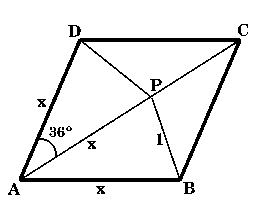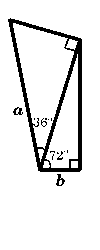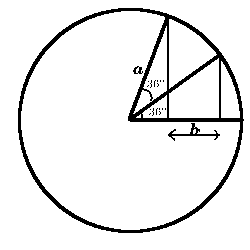#### You may also like### Pythagorean Golden Means

Show that the arithmetic mean, geometric mean and harmonic mean of a and b can be the lengths of the sides of a right-angles triangle if and only if a = bx^3, where x is the Golden Ratio.### Golden Triangle

Three triangles ABC, CBD and ABD (where D is a point on AC) are all isosceles. Find all the angles. Prove that the ratio of AB to BC is equal to the golden ratio.### Golden Powers

You add 1 to the golden ratio to get its square. How do you find higher powers?

# Gold Again

##### Age 16 to 18Challenge Level

The first part of this solution came from Dorothy, S4, Madras College, St Andrew's and the second part from Vassil, Y11, Lawnswood High School, Leeds.

Consider the rhombus as illustrated, where $x$ is an unknown length. We have to find the value of $\cos 36^\circ \cos 72^\circ .$

I filled in the remaining angles and lengths, showing triangles $PCB$ and $PCD$ to be isosceles triangles with angles of $108^\circ$, $36^\circ$ and $36^\circ$ and sides $PC = PB = PD = 1$ unit.I found $\cos 36^\circ$ and $\cos 72 ^\circ$ by using the cosine rule for triangles DCP and APD respectively. $\cos 36^\circ = {{x^2 + 1 - 1}\over 2x} = {x\over 2}$ $\cos 72^\circ = {{1 +x^2 - x^2}\over 2x} = {1\over 2x}$ Combining these two expressions, $\cos 36^\circ \cos 72^\circ = {x\over 2}.{1\over 2x}={1\over 4}.$

Consider the area of the triangle `above' the diagonal, and express it is the sum of two areas : ${1\over 2} x^2 \sin 108^\circ = {1\over 2} x\cdot1\cdot\sin 72^\circ + {1\over 2} 1\cdot 1\cdot\sin108^\circ.$ As $\sin 108^\circ = \sin 72^\circ$, this gives $x^2=x+1$ and hence $x$ is the golden ratio: $x = {1+\sqrt{5}\over 2} = \varphi .$ Hence $\cos 36^\circ - \cos 72^\circ = {x\over 2} - {1\over 2x} = {x^2-1\over 2x}={1\over 2}.$Let the common side of the two triangles be $x$. Then we have: $b = x\cdot \cos 72^\circ$ and $a= {x\over{\cos 36^\circ}}.$ Therefore ${b\over a} = \cos72^\circ \cos 36^\circ = {1\over 4}$ so $a$ is four times bigger than $b$.

 Here we have ${{x + b}\over a} =\cos 36^\circ$ and ${x\over a} = \cos 72^\circ$ so ${b\over a} = \cos 36^\circ -\cos 72^\circ = {1\over 2}.$ Therefore $a$ is twice the length of $b$.